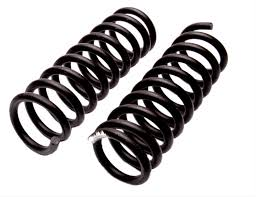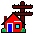# (some) Geometric Ideasextrapolations from the 1884 book Flatland (and Lineland and Spaceland) by schoolmaster Edwin Abbott Abbott

1. first imagine a single point in three dimensional space (also known as 3-d space)
• what three dimensions? These are the three spatial dimensions (x, y, z) of the Cartesian coordinate system invented by René Descartes
• this point is a mental construct with zero dimensions of its own. This is easy if we are talking about a location. This is a little more difficult if we are thinking about a pencil point where the illusion is ruined by a magnifying glass.
2. moving a single point in any direction of 3-d space will trace out a line (two end points; one line).
• line is the name of a new higher dimensional item.
3. moving a line (perpendicularly to the previous direction) will trace out a square (four points; four lines; one plane).
• square (or plane) is the name of a higher dimension item. Is the plane one surface or two? Hmmm...
4. moving a square (perpendicularly) will trace out a cube (eight points, twelve lines, 6 planes)
• cube is the name of a new higher dimensional item
5. moving a cube in 3-d space or 4-d space will trace out a hyper-cube
• many people have speculated that time can be considered a fourth dimension but we all know that time is not spatial. But Einstein's "theory of gravity" (also known as the theory of relativity) speaks of space-time as a real thing. Hmmm...
6. Related thoughts:
• Abbott describes what a citizen of flatland might see if a 3-d sphere passed through Flatland: "a point would appear; which would become a line; which would lengthen then contract back to a point before disappearing from view".
• Now think about about a single coil spring (like one of the two pictured pictured to the right) pushed through flatland: "a point would appear; then it would oscillate 10 times; then it would disappear".
• Pulling the spring back would make the oscillation appear to reverse direction.
• Pushing a spring wound in the opposite direction would also appear to reverse direction.
• I have often wondered if something similar could be adapted to properly explain Electromagnetism in our world without resorting to Maxwell's Laws
Object
Number
Object Name dimensions vertices
(points)
edges
(lines)
faces
(planes)
cubes hypercubes   dimensions vertices
(points)
edges
(lines)
faces
(plains)
cubes
Progression of a dot:             Unfolded:
1 point 0 1 - - - -   - - - - -
2 line 1 2 1 - - - point 0 2/1 - - -
3 square 2 4 4 1 - - line segments/line 1 5/2 4/1 - -
4 cube 3 8 12 6 1 - flat cross 2 14 19 (6) -
5 hypercube (Tesseract) 4 16 ? 32 ?  24 ? 8 ? 1 8 cubes on a cross 3 36 44 40/29 8
Other Stuff:
3 sided pyramid (Tetrahedron) 3 4 6 4 - - 4 triangles 2 6 9 (4) -
4 sided pyramid 3 5 8 5 - - 4 triangles, 1 square 2 8 12 (5) -
5 sided pyramid 3 6 10 6 - - 5 triangles, 1 pentagon 2 10  15 (6) -
Octahedron 3 6 12 8/(9) - - 8 triangles 2 10  17 (8) -
Dodecahedron 3 20 30 12 - - 12 triangles 2 38  49  (12) -
Icosahedron 3 12 30 20 - - 20 triangles 2 22 31 (20) -
• The Euler Formula (below) is always true for 3-d objects consisting of straight lines:
Edges = Vertices + Faces + 2
• The yellow diagonal extrapolation suggests 8 cubes may be created when a 3-d cube is moved through a fourth (time?) dimension
• Thought experiment: moving a cube from A to B in a 3-dimension space maps out lines between two cubes (one beginning; one ending) with connecting lines between the corners. A different visualization pictures a smaller cube inside a larger one with lines connecting the closest corners. When drawn out with pencil and paper you can see:
• 16 points where lines connect. The green vertical extrapolation suggests that this thought may be correct for four dimensions.
• 32 lines ((2 x 12) + 8 new ones) but this may not be valid for 4 dimensions.
• 24 planes ((2 x 6) + 12 new ones) but this may not be valid for 4 dimensions
• all 8 cubes if you search long enough.
• Observations:
• The following formula is consistent for objects 1-5:
Vertices = 2 ^ Dimensions
• Here's something I just noticed for objects 2-5:
Dimensions x Vertices / Edges = 2Back to Home
Neil Rieck